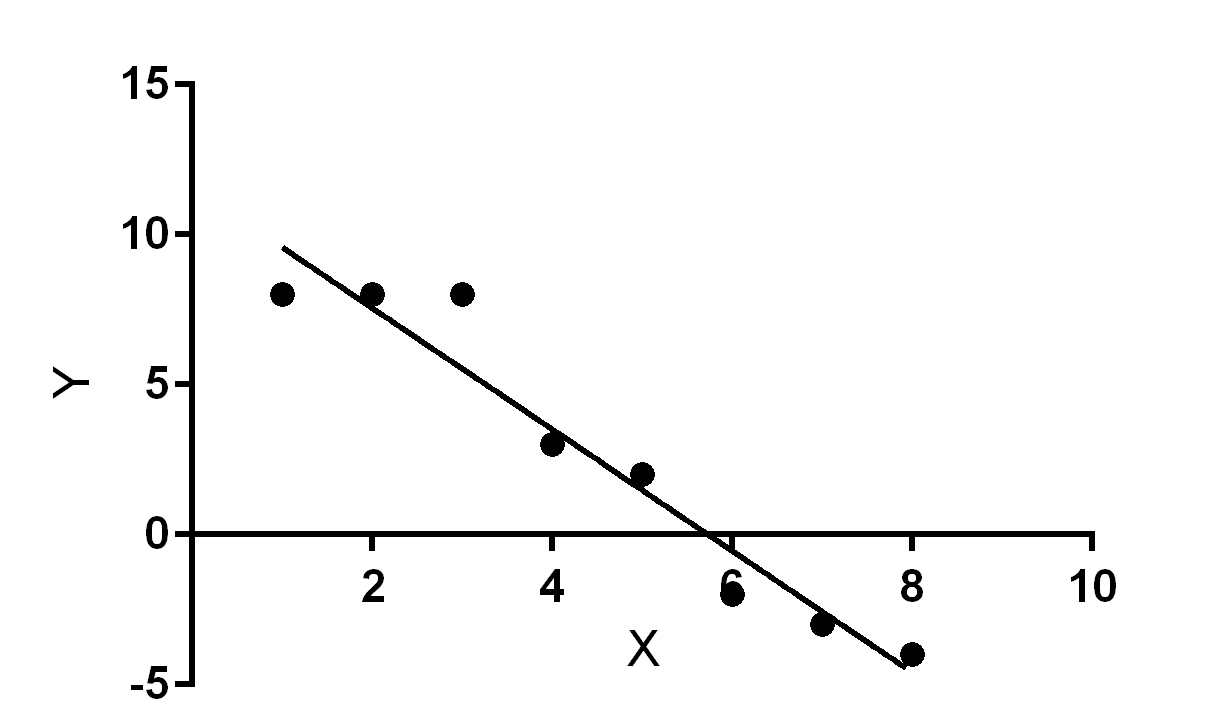# Simple linear regression with Python!

Web D.va’s foray into statistical learning begins with creating a simple linear regression calculation function in Python. Ever since I started reading about statistical learning I’ve always wanted to implement a linear regression line in code myself!

# Linear Regression

A simple linear regression model can be used to statistically predict basic things. A human can easily model some phenomenom without the computation of data. A machine, on the other hand, has to compute and calculate, using data, statistical models (such as simple linear regression) to be able to provide some value, such as a line on a two-dimensional Cartesian plane that models the relationships between some sets of variables.

## Definitions

A simple linear regression line for elementary data points has the form of $\hat{y} = a + bx$.

For a pair of a set of variables $x$ and $y$, the slope $b$ of the regression line can be defined as

$b = r_{x,y}\Big({\sigma_y \over \sigma_x}\Big)$

where $r_{x,y}$ is the correlation between the set of $x$ and $y$ variables and ${\sigma_x}$ and ${\sigma_y}$ are the standard deviations of the $x$ and $y$ set of variables. The standard deviation for a set of variables $x$ with size $n$ can be determined by calculating the square root of the variance of the set of variables, which can be determined with

$\sigma_x^2 = {1 \over n}{\sum\limits_{i=1}^n(x_i - \bar{x})^2}$

with $\bar{x}$ being the mean average of the $x$ set of variables. The correlation $r_{x,y}$ can be determined by calculating

$r_{x,y} = {\sum\limits_{i=1}^n(x_i - \bar{x})(y_i - \bar{y}) \over \sqrt{\sum\limits_{i=1}^n(x_i - \bar{x})^2 \sum\limits_{i=1}^n(y_i - \bar{y})^2}}$

with $\bar{x}$ and $\bar{y}$ being the mean average of the $x$ and $y$ sets of variables.

The $y$-intercept $a$ can be determined by $a = \bar{y} - b\bar{x}$.

By calculating the relationships between variables in a pair of sets, and thus determining relationships between data points, the statistical learning practitioner can create a model for inferring and predicting phenomenom for a machine program.

# The implementation

Using the above simple definitions, I wrote a function in Python to return the slope and $y$-intercept of the simple linear regression line for a set of data points.

I had trouble writing Python code to calculate the correlation value as I was not experienced with Python’s math facilities, so I had to resort to using an implementation of the correlation definition that was not my own. I was not familiar with how to deal with the float-int conversion type-errors that resulted from me trying to implement calculating the correlations between the variable sets.

Nonetheless, the calculateLinearRegressionLine(x, y) function returns a tuple of the slope and $y$-intercept, respectively, for the linear regression line for a set of points.

For instance, x = [1, 2, 3, 4, 5, 6, 7, 8] and y = [8, 8, 8, 3, 2, -2, -3, -4] entered as inputs to the function yield the tuple (-2.0737876550156327, 11.832044447570347) as the return value, with -2.0737876550156327 being the slope and 11.832044447570347 being the $y$-intercept of the linear regression line.

Plotted onto a graph, the resulting slope and $y$-intercept pair give a line that best fits the $x$ and $y$ data points. The new line represents the probabilities of the locations of data points with data points having the highest probability of being on or near the line.# Afterward

I chose to invest in myself by engaging in this exercise in implementing a statistical learning concept in software, the equivalent of writing your own rendering loop for a game instead of using a game framework or writing vanilla Javascript instead of using Vue.js. When I start doing machine learning projects, though, I won’t be implementing my own statistical functions, of course. I would be using something like Python’s numpy or any other statistics framework’s functions which have already implemented things like linear regression.

## What’s going to happen next?

That ultimately depends on what strategic moves I choose to make with the goal of satisfying my career objectives.

I have ideas for machine learning projects that I want to start, such as text classification and prediction. Equipped with the strength of having accomplished the completion of this linear regression exercise, I understand that I can further develop my capabilities to do machine learning.

September 30, 2017